#### 期刊菜单

Pixel-Wise Grasp Pose Detection Based on Hierarchical Network
DOI: 10.12677/CSA.2023.131008, PDF, HTML, XML, 下载: 40  浏览: 792

Abstract: In order to address the problem of estimating grasp pose under cluttered scenes using single-view image, we proposed an end-to-end pixel-wise grasp detection network based on hierarchical features. We integrated a pyramid pooling module and multi-head structure into a fully convolutional neural network to form a high-precision grasp pose detection network, effectively handling the problem of predicting grasps for unknown objects with diverse sizes and poses in clutters. The experiments showed that our proposed method significantly outperforms existing methods in Cornell grasp dataset. Physical experiments on real robotic arms are also conducted, our method achieved average grasp success rate of 88.0% at 100% completion rate in the challenging grasping task in clutter with multiple unknown objects.

1. 引言

2. 抓取位姿检测算法

2.1. 抓取位姿描述

$g=\left({g}_{p},{g}_{\theta },{g}_{w}\right)$ (1)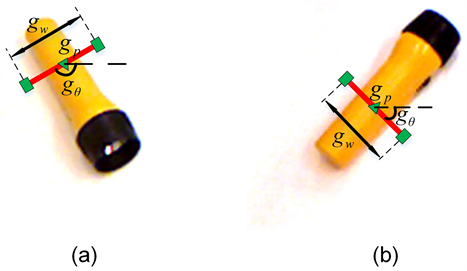Figure 1. The illustration of grasp pose representation

2.2. 网络结构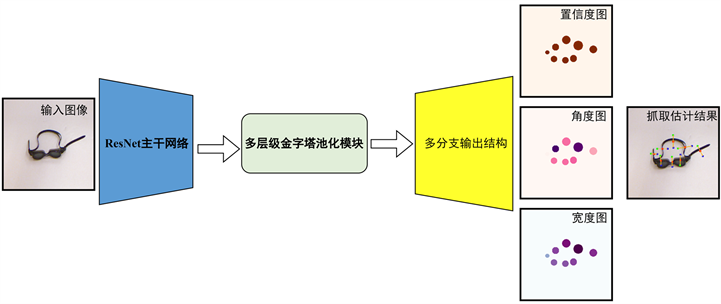Figure 2. The diagram of our proposed network architecture

2.3. 抓取图

${c\left(x,y\right)|}_{\left(x,y\right)\in {g}_{p}^{R}}={\text{e}}^{-\frac{{\left(x-u\right)}^{2}+{\left(y-v\right)}^{2}}{2{\sigma }^{2}}}$ (2)

2.4. 多层级金字塔池化模块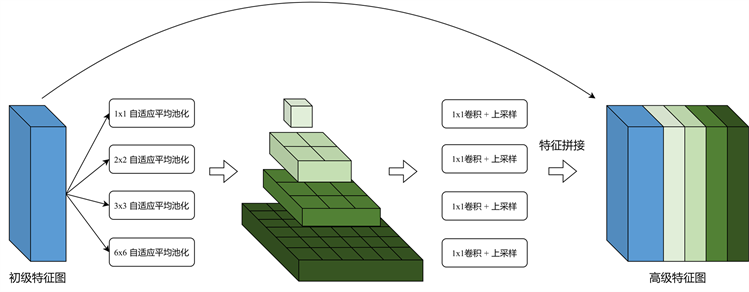Figure 3. The diagram of hierarchical pyramid pooling module

2.5. 多分支输出网络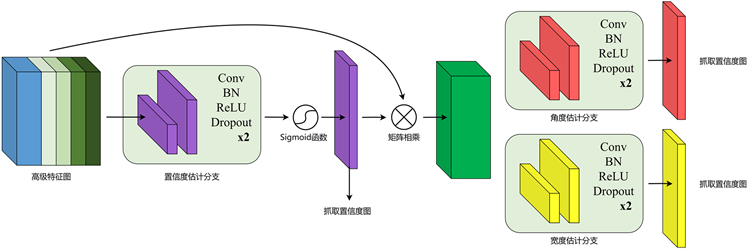Figure 4. The diagram of multi-head output structure

$\mathcal{L}\left({R}_{pos},{R}_{neg},gt,pred,l\right)=\frac{\alpha }{{N}_{pos}}\underset{{R}_{pos}}{\sum }l\left(gt,pred\right)+\frac{\beta }{{N}_{neg}}\underset{{R}_{neg}}{\sum }l\left(gt,pred\right)$ (3)

$\left\{\begin{array}{l}{\mathcal{L}}_{c}=\mathcal{L}\left({R}_{pos},{R}_{neg},{c}_{x,y},{\stackrel{^}{c}}_{x,y},{l}_{smooth}\right)\\ {R}_{pos}:x,y\in I,\text{\hspace{0.17em}}{c}_{x,y}\ge \delta \\ {R}_{neg}:x,y\in I,\text{\hspace{0.17em}}{c}_{x,y}<\delta \end{array}$ (4)

${\mathcal{L}}_{\theta }=\mathcal{L}\left({\theta }_{x,y}\in \left\{1,2,\cdots ,{N}_{A}\right\},{\theta }_{x,y}\in \left\{0\right\},{\theta }_{x,y},{\stackrel{^}{\theta }}_{x,y},{l}_{focal}\right)$ (5)

${\mathcal{L}}_{w}=\mathcal{L}\left({R}_{pos},{R}_{neg},w,\stackrel{^}{w},{l}_{smooth}\right)$ (6)

${\mathcal{L}}_{g}={\lambda }_{c}{\mathcal{L}}_{c}+{\lambda }_{\theta }{\mathcal{L}}_{\theta }+{\lambda }_{w}{\mathcal{L}}_{w}$ (7)

3. 理论实验及结果

3.1. 数据集介绍

3.2. 评估指标

1) 预测抓取角度和真实抓取角度之间的差值小于阈值 ${\delta }_{A}$，本文中 ${\delta }_{A}=\frac{\pi }{6}$

$|{g}_{\theta }-{\stackrel{^}{g}}_{\theta }|<{\delta }_{A}$ (8)

2) 当预测抓取位置和真实抓取位姿之间的Jaccard系数大于阈值 ${\delta }_{J}$，本文中 ${\delta }_{J}=0.25$

$J\left(g,\stackrel{^}{g}\right)=\frac{|g\cap \stackrel{^}{g}|}{|g\cup \stackrel{^}{g}|}>{\delta }_{J}$ (9)

3.3. 实验参数细节

3.4. 实验结果

Table 1. Experiment results on cornell dataset

${\delta }_{A}=\frac{\pi }{6}$${\delta }_{J}=0.25$ 下得到的。为了进一步验证本文网络模型性能，我们改变这两个阈值，测试网络模型在不同的阈值下准确率表现。如果在更加严格的阈值条件( ${\delta }_{A}$ 越小或 ${\delta }_{J}$ 越大)下准确率依旧维持在较高数值的话，那么可以认为网络模型具有更强的鲁棒性。我们设置从 ${\delta }_{A}=\frac{\pi }{6}$ 开始，按照固定步长不断缩小 ${\delta }_{A}$ ；从 ${\delta }_{J}=0.25$ 开始，以固定步长不断增大 ${\delta }_{J}$，从图6可以看出，我们的算法在角度阈值变小的时候保持了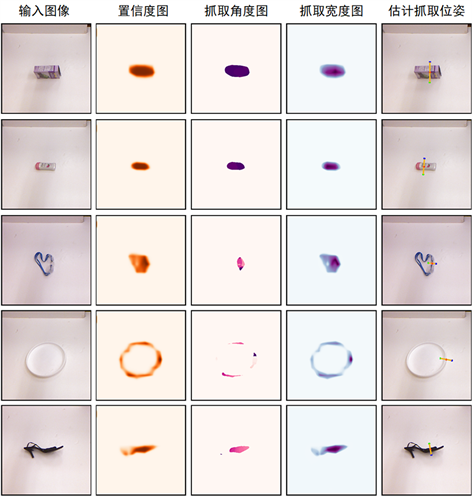Figure 5. Visualization of grasp detection results on cornell dataset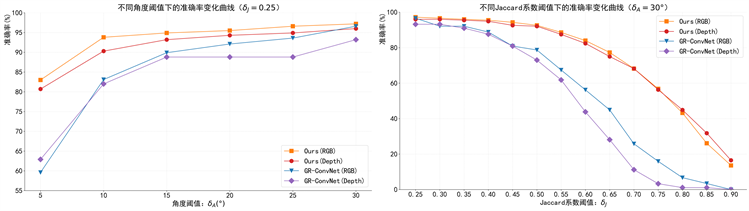Figure 6. Grasp detection of accuracy at different thresholds

4. 机械臂实物抓取实验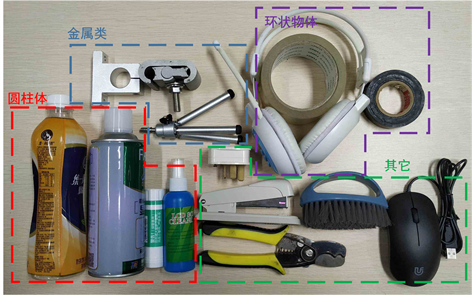Figure 7. Object candidates for robotic grasping experiments

4.1. 实验流程

4.2. 实验结果Table 2. Results of robotic grasping experiment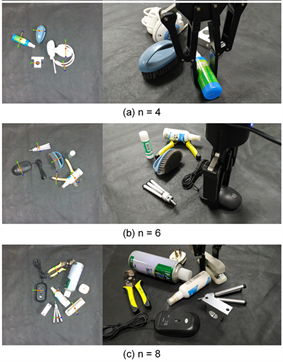Figure 8. Illustration of robotic grasping in clutter scenarios

5. 结论

  Redmon, J. and Angelova, A. (2015) Real-Time Grasp Detection Using Convolutional Neural Networks. Proceedings of the 2015 IEEE International Conference on Robotics and Automation (ICRA), Seattle, 26-30 May 2015, 1316-1322. https://doi.org/10.1109/ICRA.2015.7139361  Lenz, I., Lee, H. and Saxena, A. (2015) Deep Learning for De-tecting Robotic Grasps. The International Journal of Robotics Research, 34, 705-724. https://doi.org/10.1177/0278364914549607  Kumra, S. and Kanan, C. (2017) Robotic Grasp Detection Using Deep Convolutional Neural Networks. Proceedings of the 2017 IEEE/RSJ International Conference on Intelligent Robots and Systems (IROS), Vancouver, 24-28 September 2017, 769-776. https://doi.org/10.1109/IROS.2017.8202237  Morrison, D., Corke, P. and Leitner, J. (2018) Closing the Loop for Robotic Grasping: A Real-Time, Generative Grasp Synthesis Approach. https://doi.org/10.15607/RSS.2018.XIV.021  Morrison, D., Corke, P. and Leitner, J. (2019) Learning Robust, Real-Time, Reactive Robotic Grasping. The International Journal of Robotics Research, 39, 183-201. https://doi.org/10.1177/0278364919859066  Kumra, S., Joshi, S. and Sahin, F. (2020) Antipodal Robotic Grasping Using Generative Residual Convolutional Neural Network. Proceedings of the 2020 IEEE/RSJ International Conference on Intelligent Robots and Systems (IROS), Las Vegas, 24 October-24 January 2021, 9626-9633. https://doi.org/10.1109/IROS45743.2020.9340777  Chu, F.J., Xu, R. and Vela, P.A. (2018) Real-World Mul-tiobject, Multigrasp Detection. IEEE Robotics and Automation Letters, 3, 3355-3362. https://doi.org/10.1109/LRA.2018.2852777  Zhou, X., Lan, X., Zhang, H., Tian, Z., et al. (2018) Fully Con-volutional Grasp Detection Network with Oriented Anchor Box. Proceedings of the 2018 IEEE/RSJ International Con-ference on Intelligent Robots and Systems (IROS), Madrid, 1-5 October 2018, 7223-7230. https://doi.org/10.1109/IROS.2018.8594116  He, K., Zhang, X., Ren, S. and Sun, J. (2016) Deep Residual Learning for Image Recognition. Proceedings of the 2016 IEEE Conference on Computer Vision and Pattern Recognition (CVPR), Las Vegas, 27-30 June 2016, 770-778. https://doi.org/10.1109/CVPR.2016.90  Zhao, H., Shi, J., Qi, X., Wang, X., et al. (2017) Pyramid Scene Pars-ing Network. Proceedings of the 2017 IEEE Conference on Computer Vision and Pattern Recognition (CVPR), Honolulu, 21-26 July 2017, 6230-6239. https://doi.org/10.1109/CVPR.2017.660  Woo, S., Park, J., Lee, J.-Y. and Kweon, I.S. (2018) CBAM: Con-volutional Block Attention Module. 15th European Conference on Computer Vision, Munich, 8-14 September 2018, 1-17. https://doi.org/10.1007/978-3-030-01234-2_1  Hu, J., Shen, L., Albanie, S., Sun, G., et al. (2020) Squeeze-and-Excitation Networks. IEEE Transactions on Pattern Analysis and Machine Intelligence, 42, 2011-2023. https://doi.org/10.1109/TPAMI.2019.2913372  Yun, J., Moseson, S. and Saxena, A. (2011) Efficient Grasp-ing from RGBD Images: Learning Using a New Rectangle Representation. Proceedings of the 2011 IEEE International Conference on Robotics and Automation, Shanghai, 9-13 May 2011, 3304-3311.  Zhang, H., Lan, X., Bai, S., Zhou, X., et al. (2019) ROI-Based Robotic Grasp Detection for Object Overlapping Scenes. Proceedings of the 2019 IEEE/RSJ International Conference on Intelligent Robots and Systems (IROS), Macau, 3-8 November 2019, 4768-4775. https://doi.org/10.1109/IROS40897.2019.8967869  Song, Y., Gao, L., Li, X. and Shen, W. (2020) A Novel Robotic Grasp Detection Method Based on Region Proposal Networks. Robotics and Computer-Integrated Manufactur-ing, 65, Article ID: 101963. https://doi.org/10.1016/j.rcim.2020.101963